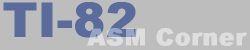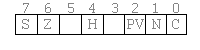Lesson 10
 TI-82 ASM Corner :: Programming Guide :: Lesson 10 Back | Home | Search
Lesson 10 : Conditional jumps and calls
In the previous lessons, we have always worked with a strict order of instructions. The programs did only one thing and always in exact the same way. In this lesson and the next one, you will learn how to make programs that evaluate user input and execute parts of the program according to this input.

Flags | Jumps and calls | Loops

FlagsTo make conditional instructions possible, the Z80 has a special register, F, which is called the flag register. Each bit (except two of them - bit 3 and bit 5) is a flag and contains 1 or 0 according to the result of the previous instruction. Please note that not all instructions affect all of the flags !
Each of the flags has its own function. For example, the zero flag will be set if the result of the last instruction was zero.
With these flags, conditional jumps and calls are possible. Special instructions allow you to jump somewhere else in your code ONLY if a flag is set or if a flag is not set.

Z-80 Flags
Bit Flag What?
0 C The carry flag will be set if the result of a calculation does not fit into a the destination register.
Take for example "ADD A,B", which adds A and B together and stores the result in A. Suppose that we load \$80 in A and \$93 in B. After "ADD A,B", the result in A will be \$13 and the carry flag will be set, because bit 9 would be 1 if there was a nineth bit.
The carry flag will also be set if a borrow is needed when subtracting. \$10 - \$20 will give you \$F0 with the carry flag set.
(C=carry set / NC=carry not set)
1 N Subtract flag, used internally by the Z80(when using BCD-instructions) so you don't have to worry about this one.
2 P/V Parity/Overflow flag. This flag has 2 functions :
- When using math instructions, the flag will be set when there is an oVerflow (flag V)
- When using logical operations, this flag is used as parity flag. It will be set if the number of set bits in the result is even (PE). When the number of set bits is odd (PO), the P flag will be reset
3 / not used
4 H Half Carry flag, used internally by the Z80 (when using BCD-instructions) so you don't have to worry about this one.
5 / not used
6 Z Zero flag, will be set when the result of an instruction is 00, or when a 00 is moved
(Z=zero / NZ=not zero)
7 S Sign flag, will be set if the result is negative (when using signed numbers). It can also be used to test if bit 7 of a result is set (see lesson 21).
(P=plus/M=minus)

Conditional jumps and calls

You already know the JP and CALL instructions from lesson 6.
But those instructions called or jumped to the function unconditional.
With the knowledge of flags, we can now extend these instructions so that conditional calls and jumps are possible too.
What is done is that a certain condition is given as an operand of the JP, JR or CALL instruction. If this condition is true (i.e. the according flag is set), the jump or call is made. If the condition is false, the program continues at the next instruction.

Conditional Calls

Here is a list of what you can do with call :

Conditional calls
Instruction Explanation
call c,Addr only call when the carry flag is set
call nc,Addr only call when the carry flag is not set
call z,Addr only call when the zero flag is set
call nz,Addr only call when the zero flag is not set
call P,Addr only call when the result was positive. (Sign flag not set)
call M,Addr only call when the result was minus. (Sign flag set)
call PO,Addr only call when Parity Odd or if there is no Overflow, according to the type of the previous instruction
call PE,Addr only call when Parity Even or if there is an Overflow, according to the type of the previous instruction

Conditional Jumps

Here's a list with the possibilities of conditional jumps.

Conditional jumps
Instruction Explanation
jp c,Addr only jump when the carry flag is set
jp nc,Addr only jump when the carry flag is not set
jp z,Addr only jump when the zero flag is set
jp nz,Addr only jump when the zero flag is not set
jp m,Addr only call when the result was negative. (Sign flag set, minus)
jp p,Addr only call when the result was positive. (Sign flag not set, plus)
jp pe,Addr only jump when Parity Even or if there is an Overflow, according to the type of the previous instruction
jp po,Addr only jump when Parity Odd or if there is no Overflow, according to the type of the previous instruction
jr c,Addr only jump when carry flag set
(relative jump)
jr nc,Addr only jump when carry flag not set
(relative jump)
jr z,Addr only jump when zero flag set
(relative jump)
jr nz,Addr only jump when zero flag not set
(relative jump)

JR and JP

Let's explain the difference between relative jumps(jr) and absolute jumps(jp).
Both can be used to jumps to a certain memory address without a condition. JR Addr and JP Addr will do exact the same thing. Where's the difference now? Well, JR can only jump 127 bytes forward and 128 bytes backwards. This way, the JR call takes only 2 bytes where a normal jump takes 3 bytes (1 opcode and a 2-byte address). There's also a difference in the speed of the instructions: JP is slightly faster than JR (in most cases).

Creating loops

With all this knowledge, we are now able to create a loop. To do this, we load the number of loops in one of the registers and decrease it at the end of the loop. If it is zero, the loop does not have to be repeated. Else, a jump is made to the beginning address of the loop.

An example:

 ``` ld a,10 ld b,0 ; initialize B to be zero Loop: inc b ; increase the contents of B with 1 dec a ; decrease the contents of A with 1 jr nz,Loop ; as long as it is not zero, keep looping ```

At the end of this little piece of code, the contents of the B register will be 10.
But the real programmers will not use this loop. Mostly the B register is used as a counter, because there is a special instruction on the Z-80 which does the dec b \ jr nz,Addr all in once. This instruction is the djnz Addr instruction.

Example:

 ``` ld b,10 ld a,0 ; initialize A to be zero Loop: inc a ; increase the contents of B with 1 djnz Loop ; dec B and Loop until B is zero ```

With these methods, you can make loops that will be executed 255 times maximum. If you want more, you will have to nest loops, because the following example won't work:

 ``` ld bc,730 ld a,0 ; initialize A to be zero Loop: inc a ; increase the contents of B with 1 dec bc jr nz,Loop ; Loop until BC is zero ```

This will give an endless loop, which you will experience as a locked up calculator. Why an endless loop? The DEC BC instruction does not affect the zero flag!

New instructions

Here is a little table with all the new instructions from this lesson.

New instructions
Mnemonic Operation
JP c,nn Conditional jump
c can be C, NC, Z, NZ, PO, PE, M or P
JR c,nn Conditional relative jump
c can be C,NC,Z or NZ
JP nn absolute jump
JR nn relative jump
DJNZ nn decrease B and relative jump